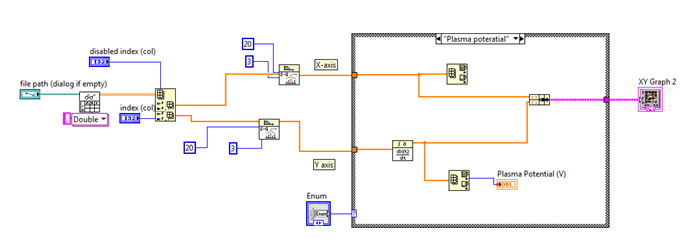# LabVIEW

cancel
Showing results for
Did you mean:

Solved!
Go to solution

## How to find the maxima of Y and its corresponding X value in XY graph.

Hi,

I am new in this field. I am getting a problem regarding to my vi and I need help.  I have read the excel data sheet in the block diagram and smoothed the X and Y values by SG filater. So my problem starts from here-

1. First I want to take derivatives of Y with respect to X . For this purpose I used the derivative function but I am not sure whether this is correct or not.

2. Next I want to plot the derivative of Y with respect to X and to find the X value for the maxima of dY/dX.

Any help will be appreciated.

Thanks-Message 1 of 9
(3,422 Views)

## Re: How to find the maxima of Y and its corresponding X value in XY graph.

@bdcpp08 wrote:

Hi,

I am new in this field. I am getting a problem regarding to my vi and I need help.  I have read the excel data sheet in the block diagram and smoothed the X and Y values by SG filater. So my problem starts from here-

1. First I want to take derivatives of Y with respect to X . For this purpose I used the derivative function but I am not sure whether this is correct or not.

2. Next I want to plot the derivative of Y with respect to X and to find the X value for the maxima of dY/dX.

Any help will be appreciated.

Thanks-can you provide exact requirement on whats your input and what is your expected output "Title and contents seems mismatching"

----------------------------------------------------------------------------------------------------------------
பழனிவேல் திருவெங்கடம்
Kidlin's Law -If you can write the problem down clearly then the matter is half solved.
-----------------------------------------------------------------------------------------------------------------
Message 2 of 9
(3,419 Views)

## Re: How to find the maxima of Y and its corresponding X value in XY graph.

use this sorting vi so that u can get the maximum of one value with respect to the other.......

There are  two ways to tell s thanks in forums : either give Kudos or  Marked Solutions

Message 3 of 9
(3,410 Views)

## Re: How to find the maxima of Y and its corresponding X value in XY graph.

Hi,

My inputs are voltage (V) and current (I) data which is in a excel spreadsheet  and my required output is the value of voltage corresponding the maxima (as shown in the picture below) of the first derivative of current with respect to the voltage (dI/dV).Message 4 of 9
(3,404 Views)

## Re: How to find the maxima of Y and its corresponding X value in XY graph.

@Rizwan00 wrote:

use this sorting vi so that u can get the maximum of one value with respect to the other.......

There are  two ways to tell s thanks in forums : either give Kudos or  Marked Solutions

are you trying to sort using some order (maximum to minimum) or trying to find the elemnt with maximum value in an array?

----------------------------------------------------------------------------------------------------------------
பழனிவேல் திருவெங்கடம்
Kidlin's Law -If you can write the problem down clearly then the matter is half solved.
-----------------------------------------------------------------------------------------------------------------
Message 5 of 9
(3,401 Views)

## Re: How to find the maxima of Y and its corresponding X value in XY graph.

use this vi... or else share excel data i will make a entire vi for ur case

There are  two ways to tell s thanks in forums : either give Kudos or  Marked Solutions

Message 6 of 9
(3,398 Views)

## Re: How to find the maxima of Y and its corresponding X value in XY graph.

i will sort and find maxima or minima

Message 7 of 9
(3,389 Views)
Solution
Accepted by topic author bdcpp08

## Re: How to find the maxima of Y and its corresponding X value in XY graph.

@bdcpp08 wrote:

Hi,

My inputs are voltage (V) and current (I) data which is in a excel spreadsheet  and my required output is the value of voltage corresponding the maxima (as shown in the picture below) of the first derivative of current with respect to the voltage (dI/dV).If my understanding is correct you can do this simple methods

From the 2D Array Extract dI/dv and Voltage Value

USe Array Min Max Function and Get the Index of Maximum value for dI/dt and use the same index in voltage array which will give you the maximum voltage value

----------------------------------------------------------------------------------------------------------------
பழனிவேல் திருவெங்கடம்
Kidlin's Law -If you can write the problem down clearly then the matter is half solved.
-----------------------------------------------------------------------------------------------------------------
Message 8 of 9
(3,384 Views)